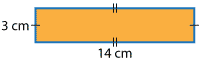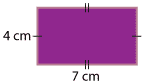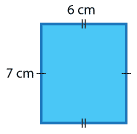### Perimeter of rectangles

On the previous page we calculated the perimeter of a rectangle by finding the sum of the lengths of its sides. In the example shown we added each side one by one. We can use the properties of a rectangle to help us calculate the perimeter more efficiently.

A rectangle has two pairs of equal sides. We can calculate the perimeter by finding the sum of the two different sides and doubling the result. The marks on the rectangles show which sides are equal in length.

 We could calculate the perimeter of the rectangle on the right:P = 3 cm + 14 cm + 3 cm + 14 cm= 34 cm Or we can use what we know about the properties of the rectangle and find the sum of the two different sides and double: P = 2 × (side one + side two)= 2 × (3 + 14)= 2 × 17= 34 cm For the rectangle on the right:P = 2 × (side one + side two)= 2 × (4 + 7)= 2 × 11= 22 cm For the rectangle on the right:P = 2 × (side one + side two)= 2 × (6 + 7)= 2 × 13= 26 cm